Next: Independence of the position Up: Probability of an evolutionary Previous: Probability of an evolutionary

#### How to compute this probability efficiently.

Let O be the point which identifies the root of the EPT. To compute the probability of a given position, we use the same idea as Dayhoff et al.  i.e. sum over all possible unknown amino acids o, w, x and y and evaluate all the mutation probabilities.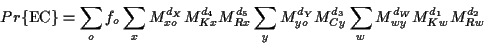The interpretation of each part of the product is simple. For each branch between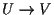we will have a term of the form MdVv u, for each unknown internal amino acid at node U we will have a sum for all its possibilities (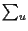) and for the root we have the sum of each possible amino acid times its natural frequency of occurrence. Lower case letters denote the particular amino acid at a given position, e.g. x is the amino acid in a given position of sequence X. The upper case K, R and C indicate the known amino acids at the leaves.

As written, this formula is very expensive to compute, it requiresproducts, where k is the number of sequences. We can reorganize this summation by the introduction of new vectors TX and SX for each internal or external node X. The value of the vector depends on the position of the root of the tree and it is computed as follows

• if X is an external leaf, i.e. a known amino acid, x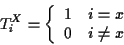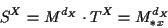(i.e. SX is just the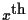column of MdX).
• if X is an internal node, then it is a ternary node. Let Y and Z be the adjacent nodes whose subtrees do not include the root of the tree (away from the root). Then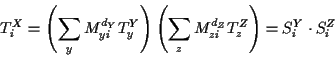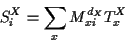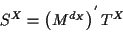These recursive definitions can be used if we do the computation from the leaves towards the root. Each S corresponding to an internal node requires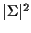multiplications, and each T requires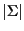multiplications. For k sequences, each of length m, this requires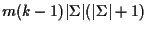multiplications (420m(k-1) for proteins), which is not inexpensive, but perfectly feasible.

The computation of the probability of the EC is done with the two immediate descendants of the root, call them X and Y.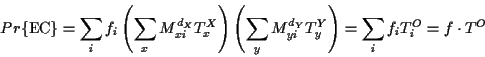The PAS at the root of the tree can be computed as follows: for each amino acid at the root compute the probability of such a configuration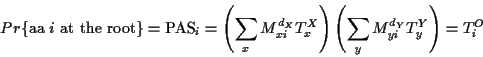where X and Y are the descendants of the root. These are probabilities of the entire EC, to find the relative probabilities for each amino acid the PAS is normalized to sum 1, i.e.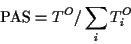Next: Independence of the position Up: Probability of an evolutionary Previous: Probability of an evolutionary
Gaston Gonnet
1998-07-14